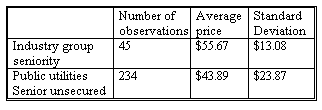### CFA Practice Question

There are 434 practice questions for this study session.

### CFA Practice Question

An analyst investigated the recovery rates on defaulted bonds by comparing recovery rates of utility bonds and other bonds stratified by seniority. The table below summarizes the findings. The group's entire sample is all senior unsecured bonds except those of utilities.The populations are normally distributed and the samples are independent. The test hypotheses are H0: μ1 - μ2 = 0 versus Ha: μ1 - μ2 ≠ 0, where μ1 is the population mean recovery rate for utilities and μ2 is the population mean recovery rate for non-utilities.

The degrees of freedom is closest to ______.

A. 15
B. 17
C. 112

df = [(171.086/45) + (569.777/234)]2 / [3.8022/45 + 2.4352/234] = 112.43

User Comment
danlan Where are 3.802 and 2.435?
PeterW2006 Hi Danlan,
(s_1)^2 / n_1 = 13.08^2 / 45 = 3.802
(s_2)^2 / n_2 = 23.87^2 / 234 = 2.435
df = (s_1^2/n_1 + s_2^2/n_2)^2 / [ (s_1^2/n_1)^2/n_1 + (s_2^2/n_2)^2/n_2 ]
= (13.08^2/45 + 23.87^2/234)^2 / [ (13.08^2/45)^2/45 + (23.87^2/234)^2/234 ]
= 112.89
kaliokale When doing H test concerning differences between means, isn't Df= N1+N2-2 ?
makp your right kaliokale BUT only if the two populations have the same variance
surob There is no need for the calculation as df (when population deviations are unequal) should not be less than 45.
chamad I thought 80 was the maximum in the table. Are you sure about 45df. may be it's saver to go with the formula. At least to double check! (If we have time)
mordja The point Surob is making is that there are 45 observations in the top sample, thus the df can be no less than 45. thus it is C.
johntan1979 112.2447

Better do the calculation because do you really think CFAI will let you run away with answer choices like these? If you practice more, you won't spend more than a minute on this.